SBOS789C August   2017  – February 2020

PRODUCTION DATA.

1. Features
2. Applications
3. Description
1.     Device Images
4. Revision History
5. Device Comparison Table
6. Pin Configuration and Functions
7. Specifications
8. Detailed Description
1. 8.1 Overview
2. 8.2 Functional Block Diagram
3. 8.3 Feature Description
4. 8.4 Device Functional Modes
9. Application and Implementation
1. 9.1 Application Information
2. 9.2 Typical Applications
1. 9.2.1 Transimpedance Amplifier
2. 9.2.2 Multichannel Sensor Interface
10. 10Power Supply Recommendations
11. 11Layout
1. 11.1 Layout Guidelines
2. 11.2 Layout Example
12. 12Device and Documentation Support
13. 13Mechanical, Packaging, and Orderable Information

• D|8
• DCN|8
• DGK|8
• DCN|8
• DGK|8

### 7.9 Typical Characteristics: VS = 24 V

at VS+ = 12 V, VS– = –12 V, RL = 1 kΩ, input and output are biased to midsupply, and TA ≈ 25°C. For AC specifications, VO = 2 VPP, G = 2 V/V, RF = 1 kΩ, and CL = 4.7 pF (unless otherwise noted)See Figure 64 and Figure 65, VO = 20 mVPP
Figure 19. Noninverting Small-Signal Frequency Response vs Gain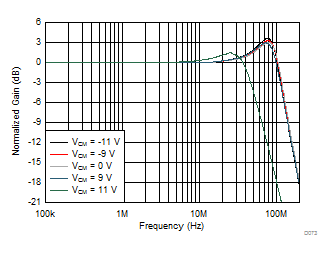See Figure 64, VO = 20 mVPP, Gain = 1 V/V, CL = 47 pF, RF = 0 Ω
Figure 21. Small-Signal Frequency Response vs Output Common-Mode Voltage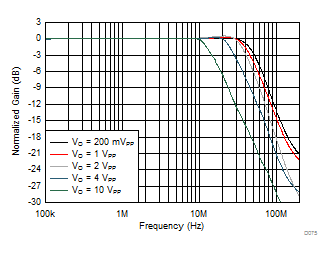See Figure 64, Gain = 2 V/V
Figure 23. Large-Signal Frequency Response vs Vo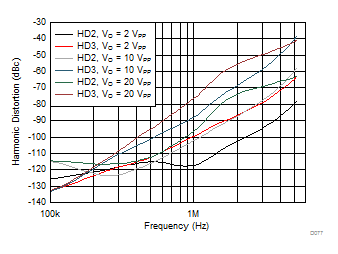See Figure 65, Gain = –1 V/V
Figure 25. Harmonic Distortion vs Frequency vs Vo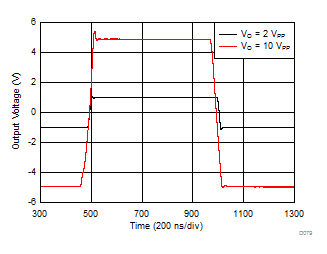See Figure 64, Gain = 1 V/V, RF = 0 Ω
Figure 27. Large-Signal Transient ResponseSee Figure 65, Gain = –1 V/V
Figure 29. Large-Signal Transient Response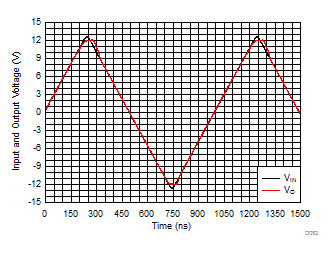See Figure 64, Gain = 1 V/V, RF = 0 Ω
Figure 31. Input Overdrive Recovery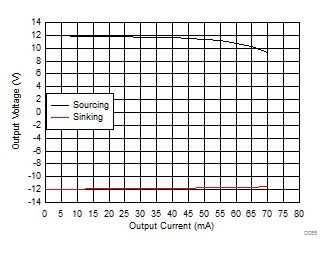Figure 33. Output Voltage Range vs Load Current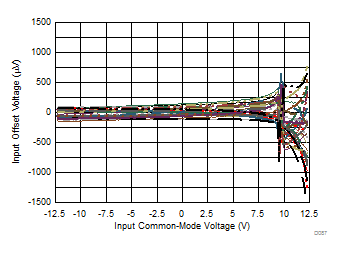Measured for 34 units
Figure 35. Input Offset Voltage vs Input Common-Mode Voltage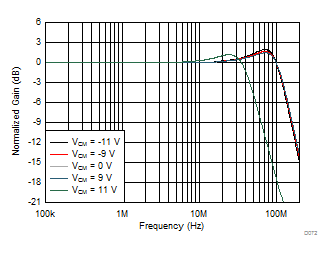See Figure 64, VO = 20 mVPP, Gain = 1 V/V, CL = 33 pF, RF = 0 Ω
Figure 20. Small-Signal Frequency Response vs Output Common-Mode Voltage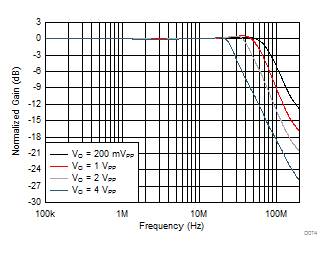See Figure 64, Gain = 1 V/V, RF = 0 Ω
Figure 22. Large-Signal Frequency Response vs Output Voltage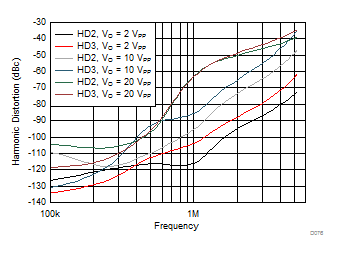See Figure 64, Gain = 2 V/V
Figure 24. Harmonic Distortion vs Frequency vs VoSee Figure 64, Gain = 1 V/V, RF = 0 Ω
Figure 26. Small-Signal Transient Response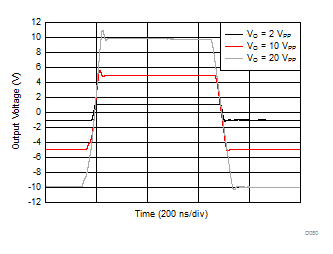See Figure 64, Gain = 2 V/V
Figure 28. Large-Signal Transient Response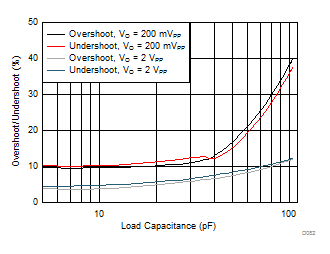See Figure 64, Gain = 1 V/V, RF = 0 Ω
Figure 30. Overshoot and Undershoot vs CL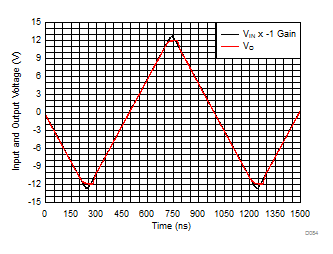See Figure 65, Gain = –1 V/V
Figure 32. Output Overdrive Recovery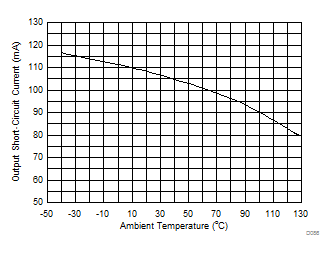Output saturated and then short-circuited, Io measured after TDelay = 5 msec
Figure 34. Output Short-Circuit Current vs Ambient Temperature